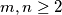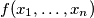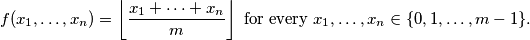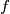### IMO Shortlist 2018 problem A6

Kvaliteta:
Avg: 0,0
Težina:
Avg: 8,0

Let$m,n\geq 2$ be integers. Let$f(x_1,\dots, x_n)$ be a polynomial with real coefficients such thatProve that the total degree of$f$ is at least$n$.

Izvor: https://www.imo-official.org/problems/IMO2018SL.pdf